# Multi-Layer Perceptron Explained: A Beginner's Guide

This article will provide a complete overview of Multi-layer perceptrons, including its history of developement, working, applications, etc.

## Introduction

Multi-layer perceptrons, or MLPs, are a powerful member of the Artificial Neural Networks family that can be used to solve complex problems that a single perceptron alone cannot. At their core, MLPs are complex and it has a collection of interconnected single perceptrons, also known as neurons or nodes, working together to process and analyze data. While this complexity can make MLPs daunting to understand, in this article we will break down this complexity and discuss the basics of MLPs. We will explore the history of its development and how the interconnected neurons and layers of the network solve most of the problems a single perceptron cannot. This article will provide a complete overview of Multi-layer perceptrons.

## What are MLPs and How it Developed?

The development of MLPs can be traced back to the 1950s when psychologist Frank Rosenblatt introduced the perceptron, a single-layer neural network (To know more about perceptron and its development check this article). However, it was soon realized that single-layer perceptrons are very limited and they could not solve some complex problems. Due to its inability to solve major problems that scientists thought, Frank's perceptron began to lose popularity, and scientists and researchers at that time almost dropped the idea of Neural Networks altogether by focusing on other machine learning algorithms

However, in the 1980s a computer scientist named Geoffrey Hinton comes up with a new idea. He noticed that the human brain is indeed a complex collection of neurons connected together and not just one. The biggest problem with perceptrons is that it is just a single-layer neural network, so he theorized that most of the limitations of perceptrons can be eliminated by stacking them together to form a multi-layer neural network, this idea proposed by Hinton leads to the emergence of a powerful algorithm, which is what we call "Multi-layer perceptron (MLP)"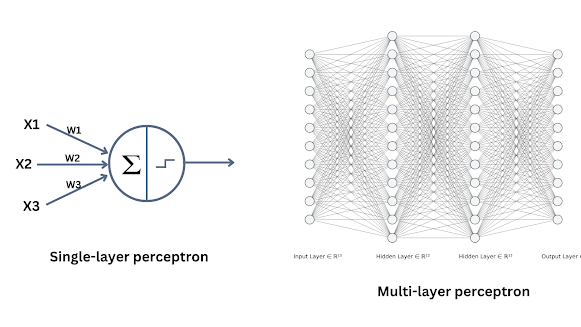Fig 1 (Single-layer vs Multi-layer perceptrons)

By definition, "A Multi-layer perceptron (MLP) is a type of artificial neural network that is composed of multiple layers of interconnected "neurons". These neurons are modeled after the neurons in the human brain, which are used to learn complex data and to make meaningful predictions".

Multi-layer perceptrons are that kind of Feed-forward network, in which the data is passed only in one direction. Unlike, some other networks like Recurrent Neural Networks, where data is passed in both directions and forms a cycle.

However, MLPs are the core algorithm behind all the powerful Neural Networks we are using today, such as CNN (Convolutional Neural Networks). In recent years, the development of deep learning techniques and the availability of large amounts of data and computational power have led to a renewed interest in MLPs. These networks are now widely used in various applications such as image recognition, natural language processing, and predictive analytics.

## Multi-layer perceptrons working

Ok, let's start with an example, Imagine a group of 7-year-old students who are working on a math problem, Imagine that each of them can only do arithmetic with two numbers. But you are giving them an equation like this 5 x 3 + 2 x 4 + 8 x 2, how can they solve it?

To solve this problem, we can break it down into smaller parts and give them to each of the students. One student can solve the first part of the equation "5 x 3 = 15" and another student can solve the second part of the equation "2 x 4 = 8". The third student can solve the third part "8 x 2 = 16".
Finally, we can simplify it to 15 + 8 + 16. Same way, one of the students in the group can solve "15 + 8 = 23" and another one can solve "23 + 16 = 39", and that's the answer

So here we are breaking down the large math problem into different sections and giving them to each of the students who are just doing really simple calculations, but as a result of the teamwork, they can solve the problem efficiently,

This is exactly the idea of how a multi-layer perceptron (MLP) works. Each neuron in the MLP is like a student in the group, and each neuron is only able to perform simple arithmetic operations. However, when these neurons are connected and work together, they can solve complex problems.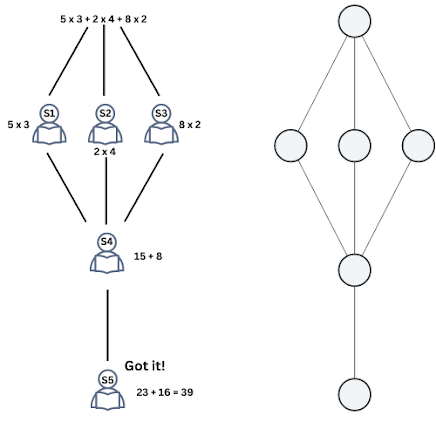Fig 2 (Example for working of MLP)

Just like how we broke down the equation into smaller parts and gave each student a specific section to solve, in an MLP, the input data is passed through different layers of interconnected neurons, each layer solving a specific part of the problem. And just like how the students combined their answers to get the final solution, the output of each neuron is passed on to the next neuron, until the final output is produced which is the solution to the complex problem.

This is just an easy example of how neural networks work, to make your mind visualize it. Neural Networks are often more versatile in solving a lot of problems, not just math problems.

### Structure of MLPs

A multi-layer perceptron (MLP) is composed of multiple layers of interconnected neurons. With our student's example, we can say that each neuron is like a student in the group, and each neuron is only able to perform simple arithmetic operations.
The structure of an MLP can be broken down into three main parts: the input layer, the hidden layers, and the output layer.
• The input layer is like the teacher giving out the math problem to the students. It receives the input data, in this case, the equation 5 x 3 + 2 x 4 + 8 x 2, and passes it on to the next layer.
• The hidden layers are like the students working together to solve the problem. Each hidden layer contains a set of interconnected neurons, which process and analyze the input data passed on from the previous layer. In this example, the hidden layer can have three neurons, each one solving a specific part of the equation "5 x 3", "2 x 4" and "8 x 2".
• The output layer is like the student who is putting together the final solution. It receives the output from the previous layers, combines them, and produces the final output which is the solution to the problem. In this example, the output neuron can be calculated as "15 + 8" and "23 + 16" to get the final result of 39.
The structure of MLP is shown here: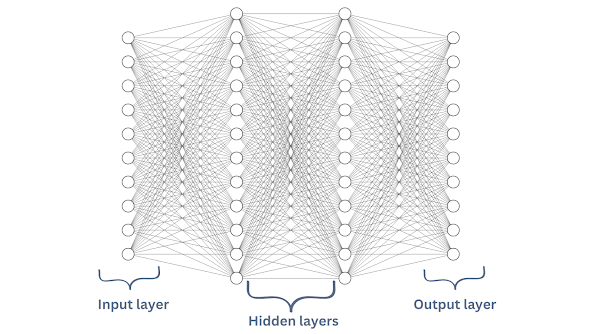Fig 3 (MLP structure)

Note that, the neurons in the input layer must be the size of the training instances, and the output layer must be the size of the output labels. However, there can be any number of neurons or layers in the hidden layer of the neural network according to the needs, So the more neurons in the hidden layer the more complex problem the network can solve.

### How data is processed inside the network?

Alright! we have discussed a good example to help you understand the structure of MLPs. Now we are going to discuss the process happening inside the network, what do the neurons actually doing, etc

As we discussed the structure of MLPs, we understand that it consists of input, hidden, and output layers.

When the data is input into the network, it is first passed through the input layer, in the input layer, there is no specific operation performed but it just transfers the input into the next layer which is the hidden layer. The neurons in the hidden layer perform some operation on the data and then it is passed to the next hidden layer if there is any. Finally, the processed data is passed to the output layer to produce the output.

In each of these neurons, we assign some weights and biases. The weights are what we call the heart of a Neural Network. Weights are basically used for determining the strength of the connection between neurons, For instance, if a neuron has a high weight, it means that it has a strong connection to the next neuron and its output will have a greater impact on the final output of the network.

On the other hand, if a neuron has a low weight, it means that it has a weaker connection to the next neuron and its output will have a lesser impact on the final output of the network.

The biases, on the other hand, are used to determine the level of activation of a neuron. A bias can be thought of as a threshold value that a neuron must reach before it produces an output.

If the input to a neuron plus its bias is greater than a certain value, the neuron will produce an output, otherwise, it will not. This allows the network to be more flexible and adaptable to different types of inputs.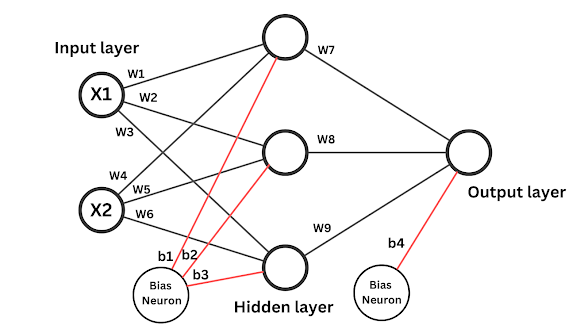Fig 4 (MLP Weights and Baises)

The computation of each of the neuron inside the network can be given by:

W = Weights of each neuron
X = inputs

This is known as the weighted sum of inputs, plus a bias. So when each neuron receives an input it will perform the weighted sum of inputs and adds a bias. The weighted sum of inputs is nothing but the dot product of the weights and inputs. This is exactly what happens inside each and every neuron inside the neural network.

If you want to see how the weighted sum of inputs is calculated, check Fig 5

But, there is something more important to note, Even if we find the weighted sum of inputs plus a bias in each case, it is nothing but purely a linear model, like Linear Regression. So if we want to do just a linear fit to the data, then why use complex Neural Networks like MLPs instead of simple Linear Regression? There is no difference between these two with this method, right?.

So here we need to bring a non-linearity to our MLP, and that is possible by introducing a non-linear function, specifically known as an Activation Function in the context of Neural Networks.

### Activation functions

You can think of activation functions as the digital equivalent of the way neurons in the brain process and respond to incoming stimuli. Just as biological neurons are activated or not activated based on the strength of the input they receive, activation functions in an MLP determine the level of activation of a neuron based on the input it receives.

The goal of activation functions is to simulate the way biological neurons process information and introduce non-linearity into the network, allowing it to learn complex relationships between inputs and outputs.

An activation function is a mathematical non-linear function that determines whether the neurons need to be activated or not. This activation function is responsible for bringing a non-linearity to the network so that it can learn really complex patterns in the given data.

So in each neuron in the network, apart from finding the weighted sum of inputs and adding a bias, these results are also passed through an activation function to produce the output of each neuron which is a non-linear value. This non-linear value is then passed to the next layer and then the process is the same.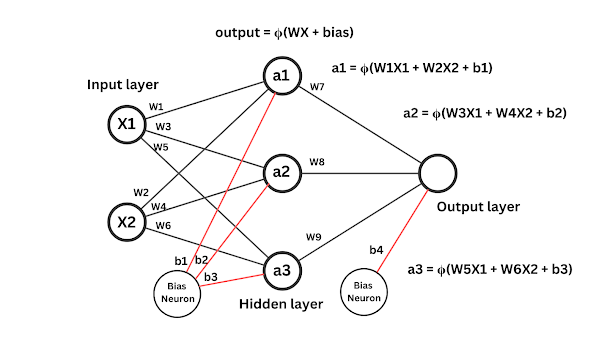Fig 5 (Weights, Biases, and activation)

So, the output of each neuron can be represented with the activation function just like this:

where 𝛟 is the Activation function.

A common activation function used in neural networks is the ReLU(Rectified Linear Unit) function.
A ReLU activation function will take the weighted sum of inputs plus the bias(XW + bias), and if the value is greater than 0, it will return the same value as the output, and if the value is less than or equal to 0, it will return 0 itself,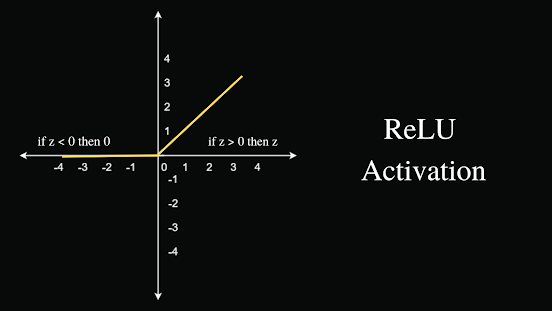Fig 6 (ReLU Activation function graph)

The mathematical expression of ReLU can be written as:

So, note that when the neuron computes the weighted sum of inputs and produces a positive value greater than 0, the ReLU fires it, and if it is 0 or a negative value, it will not get fired.

ReLU is just an example of a simple activation function in MLPs, there are a lot of activation functions in use

Some of them are:
• Sigmoid: The sigmoid function maps any input value to a value between 0 and 1. This makes it useful for modeling probability or likelihood.
• Tanh (hyperbolic tangent): The tanh function maps any input value to a value between -1 and 1. This makes it useful for modeling continuous and bounded output values.
• Softmax: The softmax function is commonly used in the output layer of a multi-class classification model. It maps the output of the final layer of a neural network to a probability distribution across all classes.
We'll surely make a dedicated article on different activation functions in Neural Networks. So be with us.

## Applications of Multi-layer Perceptron

Multi-layer perceptrons have been used in a wide variety of applications. Some of the most common applications of MLPs include:
• Image recognition: MLPs can be trained to recognize patterns in images and classify them into different categories. This is useful in applications such as facial recognition, object detection, and image segmentation.
• Natural Language Processing (NLP): MLPs can be used to understand and generate human language. This is useful in applications such as text-to-speech, machine translation, and sentiment analysis.
• Predictive modeling: It can be used to make predictions based on past data. This is useful in applications such as stock market prediction, weather forecasting, and fraud detection.
• Medical diagnosis: Can be used to diagnose diseases or interpret medical images by recognizing patterns in the data.
• Recommender systems: MLPs can be used to analyze a user's preferences and behavior to recommend products or content.

## Conclusion

In conclusion, Multi-layer perceptrons (MLPs) have proven to be a game-changer in the field of artificial neural networks. Their ability to solve complex problems through the collaboration of multiple layers of interconnected neurons, each layer solving a specific part of the problem, is truly remarkable. The use of weights and biases to adjust the strength of connections and level of activation of neurons, along with the non-linearity introduced by activation functions, has made MLPs a powerful tool for a wide range of applications. From image recognition to natural language processing, predictive modeling to robotics, and medical diagnosis to recommender systems. In fact, most of the modern types of powerful Neural Networks like CNN(Convolutional Neural Networks), RNN(Recurrent Neural Networks), GAN(Generative Adversarial Networks), etc, are inspired by the structure of MLP. So it is important to understand how MLPs work at their core,

So in the next Article, we'll discuss the learning process of MLPs. The learning process of MLPs is really simple but a little complicated at the same time. So we'll discuss in depth the popular Backpropagation algorithm and Gradient Descent on how neural networks can learn.